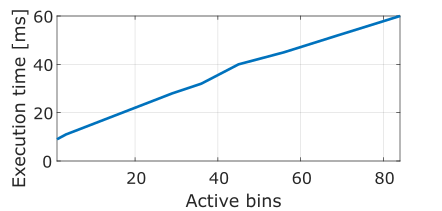Single-photon lidar is an emerging ranging technique that can obtain 3D information at kilometre distance with centimetre precision, and has important applications in self-driving cars, forest canopy monitoring, non-line-of-sight imaging and more. This modality consists of contracting a histogram of time-of-arrival of individual photons per pixel. For each object in the line-of-sight of the device there is a peak in the histogram. These peaks are found by a 3D reconstruction algorithm that takes into account the Poisson statistics of the photon-count data, while promoting spatial smoothness in the reconstructed point clouds. In a previous post, I presented an algorithm that can find multiple peaks per pixel in a matter of milliseconds even in challenging very long range scenarios with high background noise. As the algorithm needs to process the histogram data, the reconstruction time depends (linearly) on the total number of non-zero bins in the histogram:Figure Execution time of a 3D reconstruction algorithm as a function the number of non-zero bins in the collected time-of-arrival histograms

As single-photon lidar arrays get bigger and faster, the number of photons collected per histogram gets bigger, while there is an increased need for faster real-time frame rates. The volume of photon data that needs to be transmitted is ever-increasingly large, generating a data transfer bottleneck. Moreover, reconstruction algorithms are required to deal with ever-increasingly large and dense histograms, generating a computational bottleneck. So far, most attempts to alleviate these bottlenecks consisted in building coarser histograms. Despite reducing the amount of information to be transferred and processed, this approach sacrifices important depth resolution.

In (Sheehan et al., 2021), we propose a sketching method to massively compress the histograms without any significant loss of information, removing the data and computational bottlenecks. The technique builds on recent advances in compressive learning, a theory for compressing distributions. The compressed data consists of a series of $$K$$ statistics

$\Phi_k(t) = [\cos(w_k t), \sin(w_kt)]^{T} \quad \text{for} \quad k=1, \dots, K$

where $$t$$ denotes the time of arrival. The statistics can be computed on-the-fly, i.e. updated with each photon arrival, hence completely by-passing the need to construct a histogram. Below you can see the large difference between reducing the data by coarse binning the histogram and our proposed method:Figure Coarse binning and sketching

In (Sheehan et al., 2021), we propose detection methods (i.e., deciding whether there is a surface in a given pixel), which only require access to the sketched data and perform similarly to the other detection methods which require access to time-of-arrival histograms.

In (Tachella et al., 2022), we introduce a framework for using sketching together with spatially regularised reconstruction method, which can be applied to most existing spatial reconstruction methods (for example the ones in this project, and is able to massively reduce their computational complexity in mid and high photon regimes.

1.A sketching framework for reduced data transfer in photon counting lidar
IEEE Transactions on Computational Imaging 2021
2.Surface Detection for Sketched Single Photon Lidar
In 2021 29th European Signal Processing Conference (EUSIPCO) Aug 2021
3.Sketched RT3D: How to reconstruct billions of photons per second
Best Student Paper Award at ICASSP’22 Mar 2022